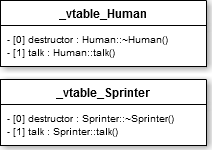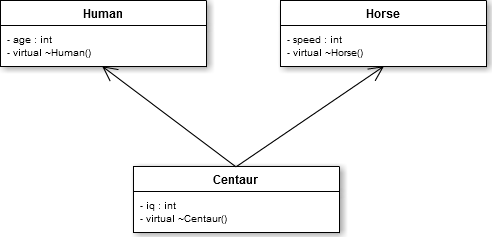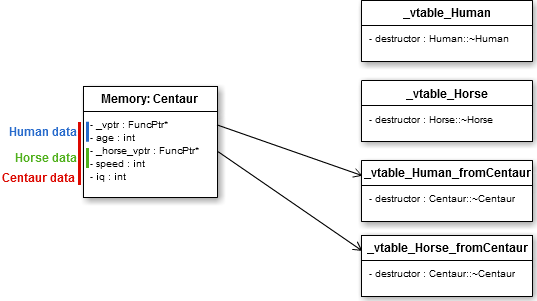\$> man42.net Blog written by a human

Here, I will explain what happens when you use the `virtual` keyword in C++.

This post will cover the following topics:

• `virtual` keyword
• `vtable` (`virtual function table` or `virtual method table`) and `vptr`
• `this` pointer and virtual functions

I will use many code examples, so don't worry if you don't understand something, look at the code example and it would be ok :)

## # Virtual keyword

When you use a pointer to a base class that was instantiated from a derived class, you can use the virtual keyword to call functions from the real instance.

``````#include <iostream>

/*
Human is the base class:
*/
class Human
{
public:
virtual ~Human()
{
}

void sayHello() const
{
std::cout << "Hello, I'm a Human!" << std::endl;
}

virtual void talk() const
{
std::cout << "Hey, how are you?" << std::endl;
}
};

/*
Sprinter is the derived class:
*/
class Sprinter : public Human
{
public:
/*
Declaring a destructor is not mandatory here as we already declared a
virtual destructor in the base class "Human" (the compiler will
automatically create a default virtual destructor).
*/
virtual ~Sprinter()
{
}

void sayHello() const
{
std::cout << "Hi, I'm a Sprinter!" << std::endl;
}

virtual void talk() const
{
std::cout << "Do you like to run?" << std::endl;
}
};

int main()
{
/*
implicit static_cast from Sprinter* to Human*:
*/
Human* fakeHuman = new Sprinter();

Human* human = new Human();

/*
"sayHello" is a non-virtual function:
internals: resolving to Human::sayHello() at compile time
output: Hello, I'm a Human!
*/
fakeHuman->sayHello();

/*
"talk" is a virtual function:
internals: resolving to Sprinter::talk() at runtime (dynamic call)
output: Do you like to run?
*/
fakeHuman->talk();

/*
"sayHello" is a non-virtual function:
internals: resolving to Human::sayHello() at compile time
output: Hello, I'm a Human!
*/
human->sayHello();

/*
"talk" is a virtual function:
internals: resolving to Human::talk() at runtime (dynamic call)
output: Hey, how are you?
*/
human->talk();

/*
Thanks to the virtual destructor declared in "Human", this object will be
destroyed properly by calling Sprinter::~Sprinter() destructor, then
the Human::~Human() destructor.
*/
delete fakeHuman;

/*
Human::~Human() is called here:
*/
delete human;

return 0;
}
``````

Ok, we understand how to use the virtual keyword, but how the compiler manages to call the correct function?!

## # vtable and vptr

A virtual function table is like a static array that contains pointers to the (virtual) functions of a class.
Each class having at least one virtual function will get its own `vtable`.

When one of these classes is instantiated, it gets an extra variable member (called `vptr`) that will contains a pointer to its `vtable`.

Here's what the `vtables` might look like:You have to know that when you compile a C++ code, the class functions are transformed into regular functions having an extra parameter: a pointer to the instance of the class, named "this" (yes, that's where the "this" pointer comes from).

So, a class function `void Human::talk();` could be transformed to a C function like `void _human_talk(Human* this);` (you can Google C++ name mangling if you are interested to know how compilers name symbols after C++ function names).

To simplify, let's use a `FuncPtr` type as a basic pointer to function: `typedef void (*FuncPtr)(void* this);`

The Human `vtable` might be:

``````static const FuncPtr _vtable_Human =
{
&_human_destructor,
&_human_talk
};
``````

The compiler just has to add an extra variable member to these classes: `private: FuncPtr const (*_vptr);`
Then initialize it in the constructor of these classes.
In the constructor of Human: `this->_vptr = &_vtable_Human;`
In the constructor of Sprinter: `this->_vptr = &_vtable_Sprinter;`

When you call a virtual function, the compiler just has to look at the vtable to call the correct function.

When you write:

``````  Human* human = new Sprinter();

human->talk();
``````

A compiler might generate this code:

``````  /*
The constructor of Sprinter will initialize the _vptr attribute:
*/
Human* human = new Sprinter();

/*
: destructor, : talk
*/
FuncPtr talkPtr = human->_vptr;

/*
Call to the correct function! Here, "human" is the "this" pointer.
It's sometimes a little bit more complicated to get a correct
"this" pointer (more on that below):
*/
(*talkPtr)(human);
``````

For your information, you can call a specific implementation of a class function by explicitly naming it:

``````  Human* human = new Sprinter();

/*
Will NOT use the vtable, and will directly call "talk"
implementation of the Human class:
*/
human->Human::talk();
``````

## #`this` pointer and virtual functions

Sometimes, the compiler has to do a little bit of arithmetic to pass the correct this pointer to a virtual function.

Imagine a class Centaur that inherits from both Human and Horse:Here is a possible memory representation of the Centaur class (note: in this example, the compiler optimizes the memory by using the same vptr attribute for both the Human and Centaur classes):So, what happens if we delete an instance of `Centaur` through a pointer to `Human`?
As the data for a `Human` and a `Centaur` starts at the same position in memory, no special operations are needed. The `this` pointer is the same for both classes.

But what happens when we use an instance of `Centaur` through a pointer to `Horse`?
Well... the static cast from a `Centaur` pointer to a `Horse` pointer will move the data pointer from some bytes (from the `Centaur` data to the `Horse` data).

``````  Centaur* centaur = new Centaur();

/*
Internally:
human = ((void*)centaur) + 0; // <= same pointer!
*/
Human* human = static_cast<Human*>(centaur);

/*
Internally:
horse = ((void*)centaur) + sizeof(Human); // <= different pointer!
*/
Horse* horse = static_cast<Horse*>(centaur);
``````

So, when you delete the "horse" pointer that is an instance of `Centaur`, how the compiler passes the correct `this` pointer?

A way to do that (the g++ way!) is to use a "wrapper" that will modify the `this` pointer then call the correct function.

``````void _destructor_horse_fromCentaur(Horse* this)
{
/*
Internally:
centaur = ((void*)this) - sizeof(Human);
*/
Centaur* centaur = static_cast<Centaur*>(this);

/*
Call to Centaur::~Centaur() then operator delete(centaur)
to free the memory.
*/
_destructor_centaur(centaur);
}
``````

The `vtable` of `Centaur` will use the `_destructor_centaur` function as destructor.
While the `vtable` of `Horse` instantiated from a `Centaur` will use the `_destructor_horse_fromCentaur` function as destructor.

I hope this explanation helped you to understand how the "magic" of C++ really works!
Please tell me if I made some mistakes or if something is unclear. Thank you!